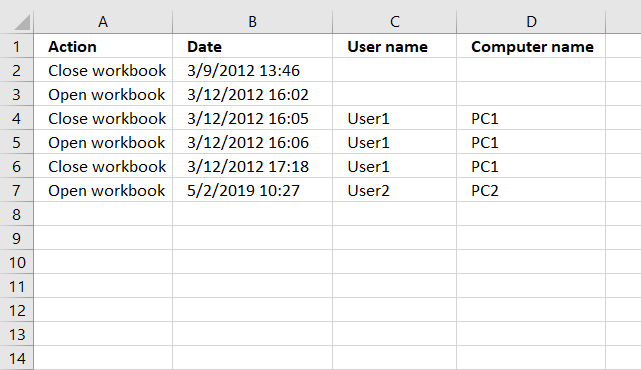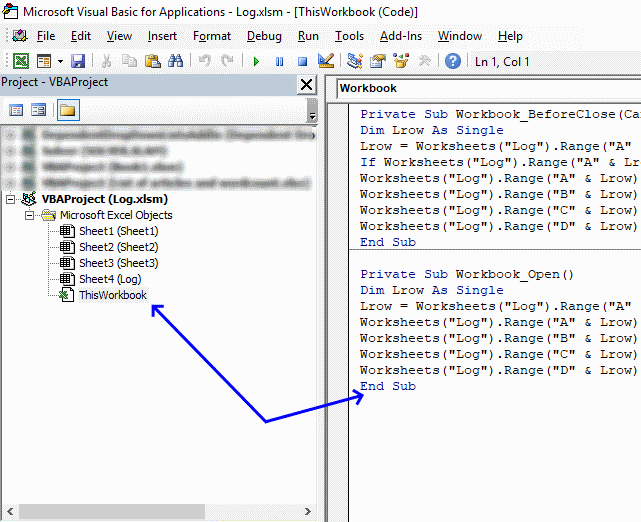Author: Oscar Cronquist Article last updated on May 02, 2019This article demonstrates how to automatically create log entries when a workbook opens or closes using event code. Column A contains the action, Open or Close, column B contains the date and time.

Column C contains the user name and column D contains the computer name. Do not paste the code below to a regular module, that won't work. There are detailed instructions below.

VBA code

```'This event code begins right before the user closes the workbook.
Private Sub Workbook_BeforeClose(Cancel As Boolean)

'Dimension variable and declare data type
Dim Lrow As Single

'Save the row of the first empty cell in column A to variable Lrow.
Lrow = Worksheets("Log").Range("A" & Rows.Count).End(xlUp).Row + 1

'Check if cell above equals text value "Close Workbook",
'if true then withdraw value in Lrow with 1 and then save the result to Lrow
If Worksheets("Log").Range("A" & Lrow - 1).Value = "Close workbook" Then Lrow = Lrow - 1

'Save text value "Close Workbook" to cell
Worksheets("Log").Range("A" & Lrow).Value = "Close workbook"

'Save date and time to the corresponding cell in column B.
Worksheets("Log").Range("B" & Lrow).Value = Now
End Sub
```
```'This event code starts when the workbook is opened.
Private Sub Workbook_Open()

'Dimension variable and declare data type
Dim Lrow As Single

'Save the row of the first empty cell in column A to variable Lrow.
Lrow = Worksheets("Log").Range("A" & Rows.Count).End(xlUp).Row + 1

'Save text value "Open Workbook" to the first empty cell
Worksheets("Log").Range("A" & Lrow).Value = "Open workbook"

Save date and time to the corresponding cell in column B.
Worksheets("Log").Range("B" & Lrow).Value = Now
End Sub
```

### Where to copy code?1. Go to tab "Developer" on the ribbon and press with left mouse button on "Visual Basic" button to open the Visual Basic Editor or press shortcut keys Alt+F11
2. Double press with left mouse button on "This workbook" in the project explorer.
3. Paste code to workbook module.